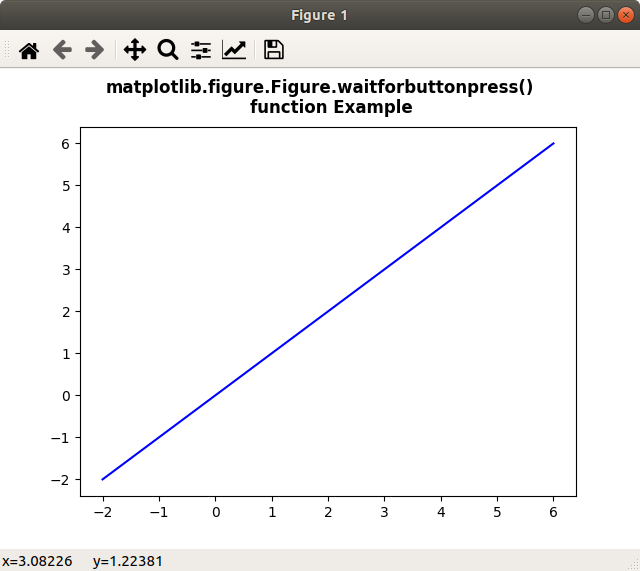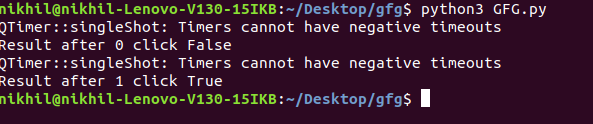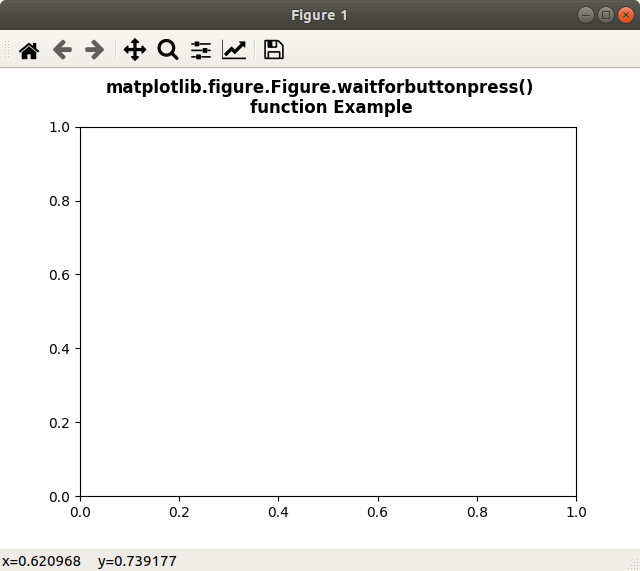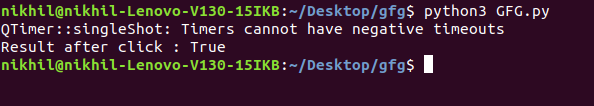Related Articles

# Matplotlib.figure.Figure.waitforbuttonpress() in Python

• Last Updated : 03 May, 2020

Matplotlib is a library in Python and it is numerical – mathematical extension for NumPy library. The figure module provides the top-level Artist, the Figure, which contains all the plot elements. This module is used to control the default spacing of the subplots and top level container for all plot elements.

## matplotlib.figure.Figure.waitforbuttonpress() method

The waitforbuttonpress() method figure module of matplotlib library is used for blocking call to interact with the figure.

Syntax: waitforbuttonpress(self, timeout=-1)

Parameters: This method accept the following parameters that are discussed below:

• timeout: This parameter is the timeout value.

Returns: This method does not returns any value.

Below examples illustrate the matplotlib.figure.Figure.waitforbuttonpress() function in matplotlib.figure:

Example 1:

 `# Implementation of matplotlib function``import` `numpy as np``import` `matplotlib.pyplot as plt`` ` `for` `ite ``in` `range``(``2``):``    ``x ``=` `np.linspace(``-``2``, ``6``, ``100``)``    ``y ``=` `(ite ``+` `1``)``*``x`` ` `    ``fig ``=` `plt.figure()``    ``ax ``=` `fig.subplots()``    ``ax.plot(x, y, ``'-b'``)`` ` `    ``fig.suptitle(``"""matplotlib.figure.Figure.waitforbuttonpress()``    ``function Example\n\n"""``, fontweight ``=``"bold")`` ` `    ``w ``=` `fig.waitforbuttonpress()``    ``print``(``"Result after"``, ite, ``"click"``, w)`` ` `    ``fig.show()`

Output:Example 2:

 `# Implementation of matplotlib function``import` `numpy as np``import` `matplotlib.cm as cm``import` `matplotlib.mlab as mlab``import` `matplotlib.pyplot as plt`` ` `fig ``=` `plt.figure()``ax ``=` `fig.subplots()`` ` `def` `tellme(s):``     ` `    ``fig.suptitle(s, fontweight ``=``"bold"``)``    ``fig.canvas.draw()``    ``renderer ``=` `fig.canvas.renderer``    ``fig.draw(renderer)`` ` `fig.clf()``ax.axis([``-``1.``, ``1.``, ``-``1.``, ``1.``])``plt.setp(plt.gca(), autoscale_on ``=` `False``)`` ` `tellme(``"""matplotlib.figure.Figure.waitforbuttonpress()``    ``function Example\n\n"""``)`` ` `w ``=` `fig.waitforbuttonpress()``print``(``"Result after click :"``, w)``fig.show()`

Output:Attention geek! Strengthen your foundations with the Python Programming Foundation Course and learn the basics.

To begin with, your interview preparations Enhance your Data Structures concepts with the Python DS Course. And to begin with your Machine Learning Journey, join the Machine Learning – Basic Level Course

My Personal Notes arrow_drop_up